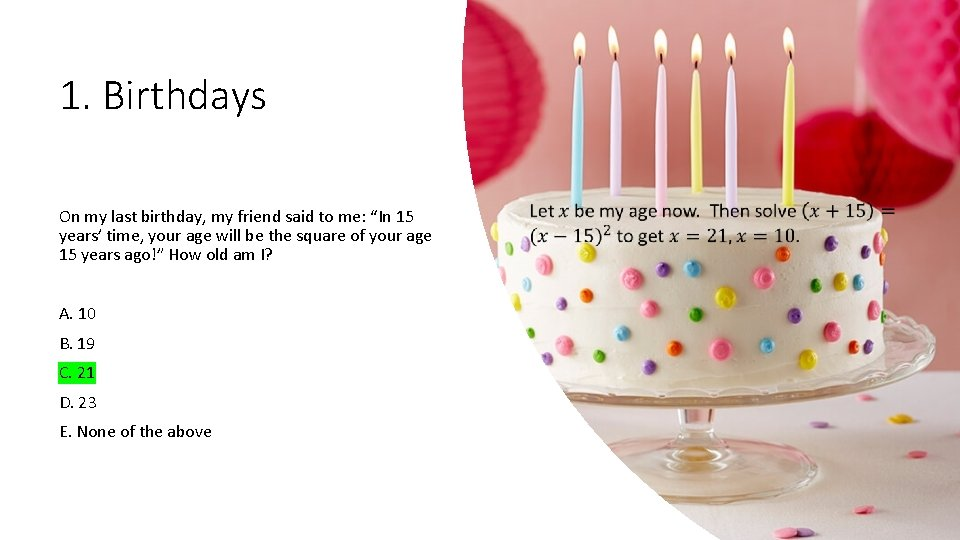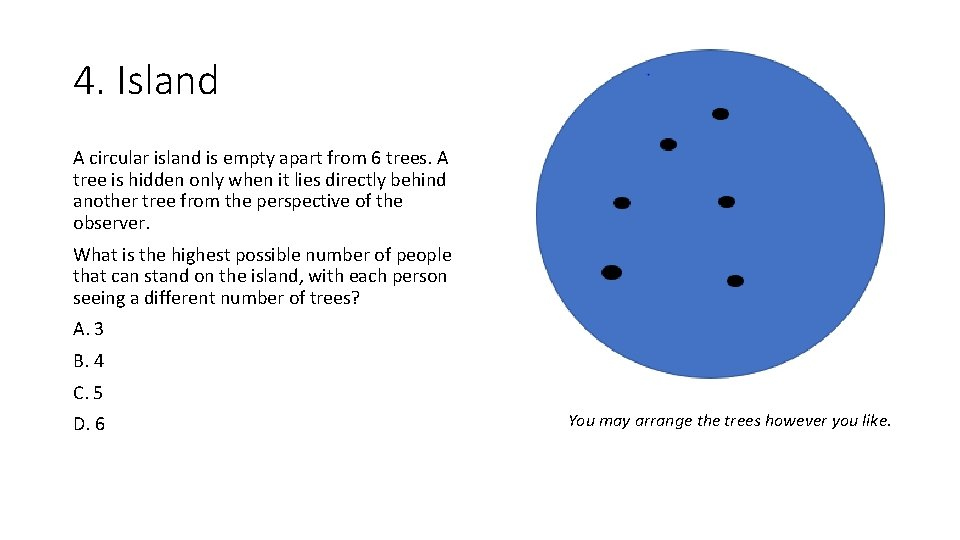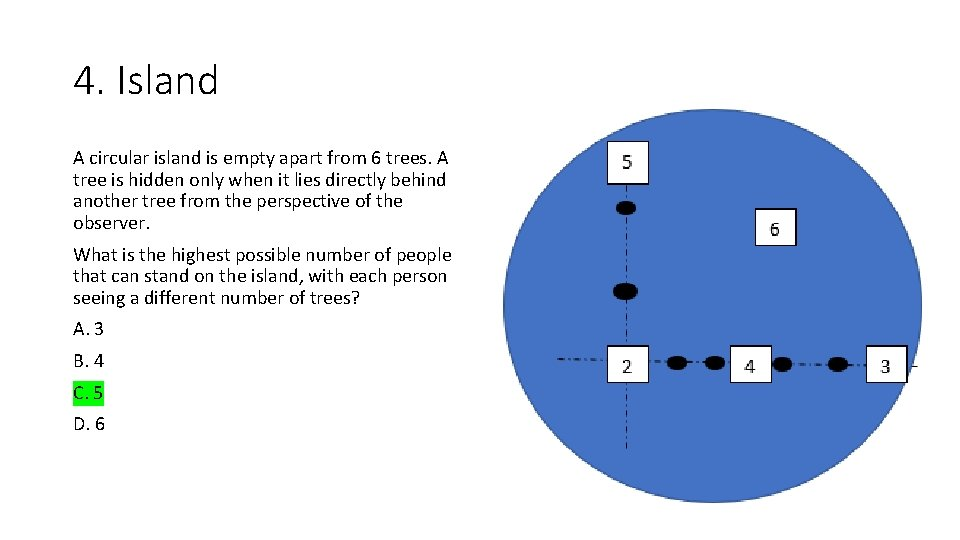# The Answers Quiz 1 Birthdays On my last

• Slides: 91. Birthdays On my last birthday, my friend said to me: “In 15 years’ time, your age will be the square of your age 15 years ago!” How old am I? A. 10 B. 19 C. 21 D. 23 E. None of the above1. Birthdays On my last birthday, my friend said to me: “In 15 years’ time, your age will be the square of your age 15 years ago!” How old am I? A. 10 B. 19 C. 21 D. 23 E. None of the above2. Snack time Break a breadstick in two places, what is the probability that you can make a triangle with the remaining pieces? A. 1/4 B. 1/3 C. 1/6 D. 1 E. None of the above2. Snack time Break a breadstick in two places, what is the probability that you can make a triangle with the remaining pieces? A. 1/4 B. 1/3 C. 1/6 D. 1 E. None of the above A triangle is only possible if no section is larger than ½. Both breaks are on the same side of the midpoint with probability ½. If the breaks a and b are on different sides of the midpoint then the probability that a is further left in its half than b is in the right half is ½. Put these together to get the answer ¼.3. Old Ghizas Pharaoh Matheses built a square based pyramid filled with gold so that four sides are equilateral triangles and the base is a square. Pharaoh Matheses’ son, Bob, squandered a lot of gold in his lifetime. He took the four equilateral triangles from Matheses’ pyramid and built a tetrahedron (triangle based pyramid) filled with gold. How much gold does Bob’s pyramid contain as a fraction of the gold that his father’s pyramid contained? A. 1/2 B. 1/3 HINT: The volume of pyramid is 1/3 × Height × Area of the base C. 3/4 D. 1/6 E. None of the above3. Old Ghizas A. 1/2 B. 1/3 C. 3/4 D. 2/3 E. None of the above4. Island A circular island is empty apart from 6 trees. A tree is hidden only when it lies directly behind another tree from the perspective of the observer. What is the highest possible number of people that can stand on the island, with each person seeing a different number of trees? A. 3 B. 4 C. 5 D. 6 You may arrange the trees however you like.4. Island A circular island is empty apart from 6 trees. A tree is hidden only when it lies directly behind another tree from the perspective of the observer. What is the highest possible number of people that can stand on the island, with each person seeing a different number of trees? A. 3 B. 4 C. 5 D. 6